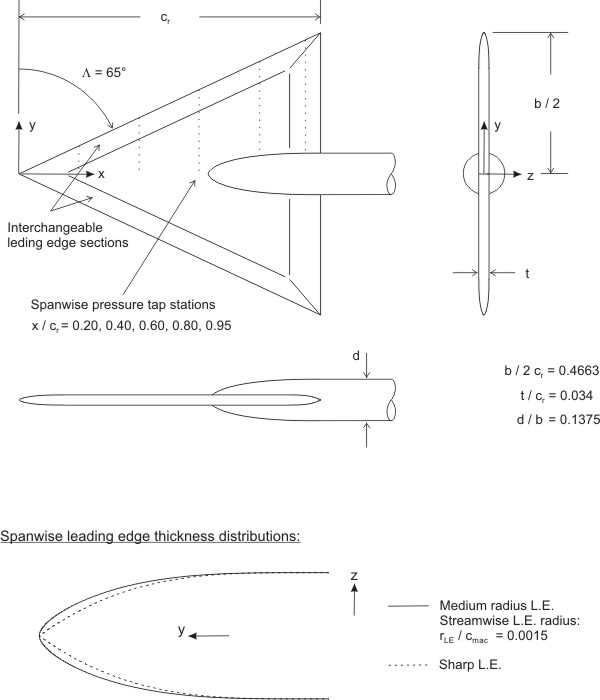# Vortex breakdown above a delta wing with sharp leading edge

## Introduction

This test case is concerned with the prediction of vortex breakdown above a delta wing with a sharp leading edge at high angle of attack. The main modelling issue is the prediction of the location of the breakdown of the primary vortex above the wing. This primary vortex is formed as the shear layer emanating from the leading edge rolls up. Thus, it may be expected that the vortex breakdown is sensitive to the instabilities developing in the shear layer. A second modelling issue for this case is therefore the prediction of shear layer instabilities. A third, minor modelling issue is pressure-induced separation: even though the primary separation is fixed by the sharp leading edge, secondary pressure-induced separation may occur between the primary vortex and the leading edge.

For this test case, the NASA delta wing geometry of Chu and Luckring (1996) with a sharp leading edge and a leading-edge sweep angle of ${65^{\circ }}$is considered. This geometry was also used in the Second International Vortex Flow Experiment (VFE-2, http://www.dlr.de/as/VFE-2). A large set of experiments and numerical results is available, see the Summary Report of the NATO RTO Task Group AVT-113 (RTO TR-AVT-113, chapter 17--34, 2009). The most detailed experiments within VFE-2 are the experiments of TU München (Furman and Breitsamter, 2008 and 2009), which include steady and unsteady measurements of pressure and velocity. These experiments of TU München are considered here.

In the EU-project ATAAC CFD computations were performed belonging to the family of Detached Eddy Simulation (DES). DES is a hybrid RANS--LES approach in which the attached boundary layers are modelled with RANS while the (strongly) separated flow regions are modelled with LES. One of the difficulties of this type of method consists of the development of resolved turbulence as the method switches from RANS to LES. For the present test case, this concerns the development of instabilities in the shear layers. As will be shown, the standard DES approach does not suffice, but modifications are necessary to induce a more rapid development of shear-layer instabilities and full 3D turbulence.

In the EU-project DESider (Haase et al., 2007), DES and SAS computations were performed for the vortex breakdown above a delta wing with a sharp leading edge (Ceresola, 2009). A different wing with a higher leading-edge sweep (${76^{\circ }}$) was used. Satisfactory results were obtained for the time-averaged quantities, in particular in terms of the location of the vortex breakdown and the strength of the secondary separation. The prediction of fluctuating quantities, such as the RMS values of pressure and velocity components, however, was less satisfactory, showing a larger variation between the partners and a significant deviation from the experiment. To improve the prediction of fluctuating quantities, it was suggested to employ a more isotropic grid in the area of the primary vortex. This suggestion has been followed here.

1. {ATAAC project (Advanced Turbulence Simulation for Aerodynamic Application Challenges), funded by the European Union under Grant Agreement no.~233710
2. DESider project (Detached Eddy Simulation for Industrial Aerodynamics) funded by the European Union under Contract No.~AST3-CT-2003-50284 of the European Commission

## Relevance to Industrial Sector

The wings of fighter aircraft as well as certain UAVs typically have a delta shape. The lift generated by such wings is, to a large extent, determined by the suction due to the main vortex above the wing. When the vortex breaks down, it generates a much weaker suction and much of the generated lift is lost. For the prediction of aircraft performance, it is therefore important to be able to compute the strength and location of vortex breakdown as well as the flow conditions at which breakdown occurs.

## Design or Assessment Parameters

The parameters used to judge the CFD computations are

• the time-averaged pressure coefficient ${\langle C_{p}\rangle }$and the root-mean-square (RMS) value of the pressure coefficient ${C_{p,{\text{rms}}}=(\langle C_{p}^{2}\rangle -\langle C_{p}\rangle ^{2})^{1/2}}$, both at the wing surface, with the instantaneous pressure coefficient given by ${C_{p}=(p-p_{\infty })/({\frac {1}{2}}\rho _{\infty }u_{\infty }^{2})}$,
• the dimensionless time-averaged velocity vector ${\langle {\vec {u}}\rangle /u_{\infty }}$at several wing cross sections, and
• the dimensionless resolved turbulent kinetic energy ${k/u_{\infty }^{2}={\frac {1}{2}}(\langle |{\vec {u}}|^{2}\rangle -|\langle {\vec {u}}\rangle |^{2})/u_{\infty }^{2}}$at several wing cross sections,

with the brackets ${\langle f\rangle }$indicating the time average of ${f}$.

## Flow Domain Geometry

The NASA delta wing geometry has a ${65^{\circ }}$leading-edge sweep and a sharp leading edge. The geometry includes a sting. The analytic definition of the geometry (including sting) is given by Chu and Luckring (1996). A sketch of the geometry is given in Figure 1, with the origin of the coordinate system located at the apex of the wing, i.e., the most upstream vertex. In the experiment of München, a root chord ${c_{r}=0.98}$m has been used. A discretized definition of the geometry wing.dat is available, with the coordinates scaled by the root chord ${c_{r}}$.

Figure 1: Geometry of VFE2 delta wing (taken from Chu and Luckring, 1996)## Flow Physics and Fluid Dynamics Data

The experiment has been performed at two Mach numbers (${M=0.07}$and ${0.14}$) with corresponding Reynolds number ${Re_{\text{mac}}=1\cdot 10^{6}}$and ${2\cdot 10^{6}}$) and at three angles of attack (${\alpha =13^{\circ }}$, ${18^{\circ }}$, and ${23^{\circ }}$). Vortex breakdown occurs at the highest angle of attack, and the most extensive experimental data set is available for the lowest Mach number, including velocity fluctuations. Therefore, the flow conditions considered here are

${M=0.07,\quad Re_{\text{mac}}=1\cdot 10^{6},\quad \alpha =23^{\circ }.}$Note that the Reynolds number is based on the mean aerodynamic chord ${c_{\text{mac}}={\frac {2}{3}}c_{r}}$.